The Missionaries and Cannibals Problem (MCP) is a classic river-crossing logic puzzle that derives from the famous Jealous Husbands problem. The earliest version of the MCP problem was described by Pocock in 1891. In the article “The jealous husbands and the missionaries and cannibals” issued by Ian Pressman and David Singmaster on The Mathematical Gazette73 ( JSTOR 3619658), the following theorem was stated as the 4th theorem without proof for this river crossing problem:

THEOREM 4. The minimal number of crossings to ferry n >= 3 missionaries and n cannibals across a river with an island, using a two-person boat and bank-to- bank crossings, is 4n - 1.

The Missionaries and Cannibals Problem is usually defined as follows:

On one bank of a river are 3 missionaries and 3 cannibals. There is 1 boat available that can carry at most 2 people and that they would like to use to cross the river. If the cannibals ever outnumber the missionaries on either of the river’s banks or on the boat, the missionaries will get eaten. How can the boat be used to carry all the missionaries and cannibals across the river safely? The boat cannot cross the river by itself with no people on board and there is no island in the middle of the river.

### Solving the problem

To build a system to solve this problem, we can define how to represent the state of the system and how the states will change from the actions applied. We also need to define the initial state and the final state, so the problem solving is abstracted as finding a path from the initial state to the final state. Through this method, we can solve the problem with the help of computer graph theory knowledge to find a connected one-way graph path. The detailed algorithm can be described as such:

1. The primary argument for the system is the number of Missionaries (M), the number of Cannibals (C) and the capacity of the boat (B). For the upper problem, the M=3, C=3 and B=2.
2. The system state can be uniquely defined by the state of missionaries, cannibals and the boat on the left bank. In other words, [m=3, c=3, b=1] indicates there are 3 missionaries, 3 cannibals and a one-person boat on the left bank. For the state of the other bank, it’s uniquely determined by the left bank after crossing. Both banks need to always abide by the game's rules for all [m, c, b], m>=0, c>=0, m>=c if m>0.
3. The number of valid crossing actions depends on the capacity of the boat and the state of the departure ferry. If the capacity of a boat is 2, the possible states of the boat need to meet all of the following conditions of the rules defined in #2:
• (p+q)<=B : a boat can carry at most B people.
• (p=0 OR (p>0 AND p>=q)): cannibals can’t outnumber the missionaries on the boat if there is any missionary.
• NOT (p=0 AND q=0): the boat cannot cross the river by itself with no people.

So, we get following actions table for river crossing:

 (p, q) 0 1 2 0 (0, 0) (0, 1) (0, 2) 1 (1,0) (1,1) (1,2) 2 (2,0) (2,1) (2,2)

1. Starting from the initial system state, we can use Breadth First Search (BFS) algorithm to drive state space transition. The starting point is the initial state, while the end point is the target state. To avoid reentrant visits, we need to use a dictionary to record the nodes that have been visited. We also need a queue to pool the newfound system state which has not been visited yet. So, we can apply the actions defined in #3 until the state space is traversed.
2. The output of #4 is the path segment for a final solution. Whenever we find a solution, we need to dump out the full path. And all these paths form a Directed Acyclic Graph (DAG). For those endpoints that are not the final goal state, we need to remove them to build a single clean graph with the final state as the endpoint.

### Visualizing the solution

The following result is generated by the upper algorithm implementation by SAS for the MCP problem (M=3, C=3 and B=2). It’s a directed acyclic graph that can represent all possible solutions on one page. The NETDRAW procedure in SAS was designed to draw a network diagram of the activities in a project, but we use it to visualize nodes and relationships for a directed acyclic graph (DAG) here (click here to download the precompiled code).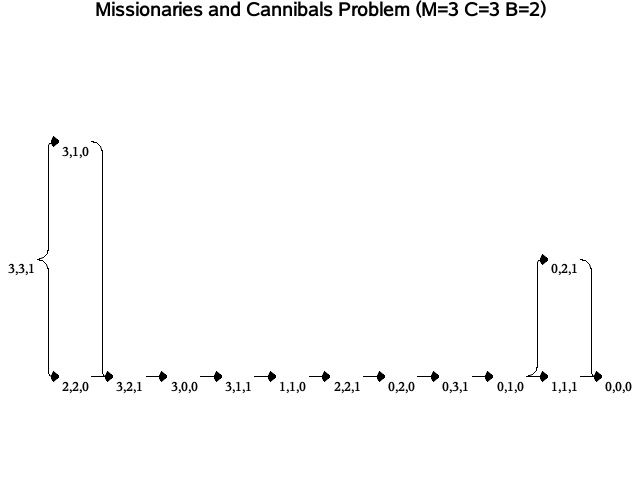We also can generate the step description for a solution (top-most path) in that directed acyclic graph. For example, the first intuitive solution for (M=3, C=3, B=2) is listed below. It is one of the 4 possible solutions revealed by the upper directed acyclic graph. There are three other variants for (M=3, C=3 and B=2) besides the following solution.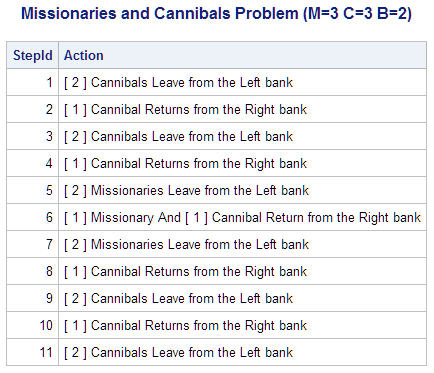Generally, if the boat's capacity is 2 (B=2) and the number of missionaries and cannibals is equal (C=M), we draw the following conclusions:

• When M = 1, there is one and only one solution, that is, N(M=1, C=1, B=2) =1.
• When M = 2, there are 4 different solutions, that is, N(M=2, C=2, B=2) =4. Each solution needs 5 trips.
• When M = 3, there are 4 different solutions, that is, N(M=3, C=3, B=2) =4. Each solution needs 11 tips. In fact, this is the only case meet the 4n-1 statement of THEOREM #4.
• When M>=4, there is no solution, that is, N(M>=4, C=M, B=2)=0.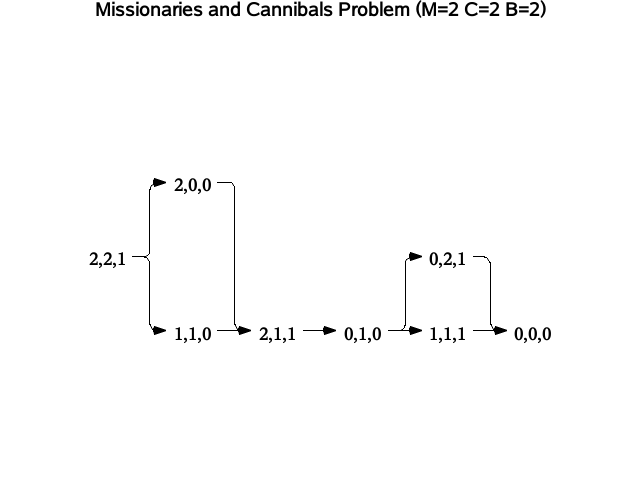When the number of cannibals is less than that of missionaries, such as 1 less, that is (C=M-1), all values of M have solutions. This is because fewer cannibals weaken the constraints, so there will be more solutions.

• When M = 1, there is one and only one solution, that is, N(M=1, C=0, B=2)=1.
• When M = 2, there are 3 different solutions, that is, N(M=2, C=1, B=2)=3. Each solution needs 3 trips.
• When M = 3, there are 9 different solutions, that is, N(M=3, C=2, B=2) =9. Each solution needs 7 trips.
• When M = 4, there are 25 different solutions, that is, N(M=4, C=3, B=2)=25. Each solution needs 11 trips.

If the number of missionaries and cannibals is equal (C=M) when the boat's capacity is 3 (B=3), then:

• When M = 1, there is one and only one solution, that is, N(M=1, C=1, B=3) = 1.
• When M = 2, there are 5 different solutions, that is, N(M=2, C=2, B=3) = 5. Each solution needs 3 trips.
• When M = 3, there are 6 different solutions, that is, N(M=3, C=3, B=3) = 6. Each solution needs 5 trips.
• When M = 4, there are 32 different solutions, that is, N(M=4, C=4, B=3) = 32. Each solution needs 9 trips.
• When M = 5, there are 25 different solutions, that is, N(M=5, C=5, B=3) = 25. Each solution needs 11 trips.
• When M>=6, there is no solution, that is, N(M>=6, C=M, B=3) = 0.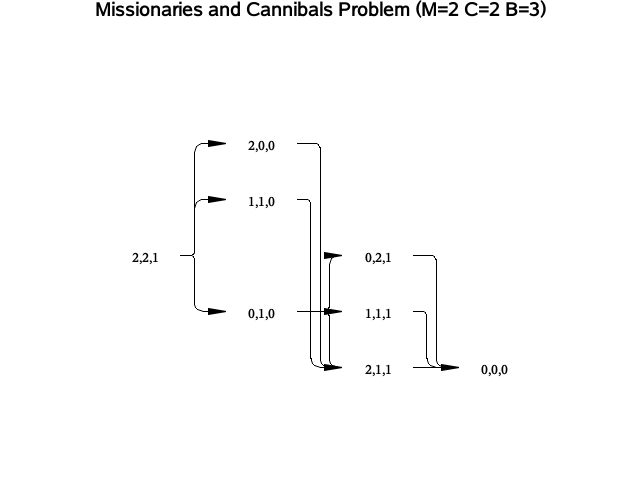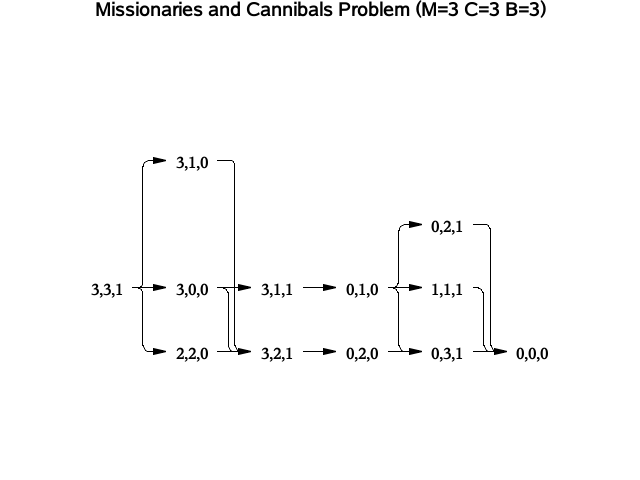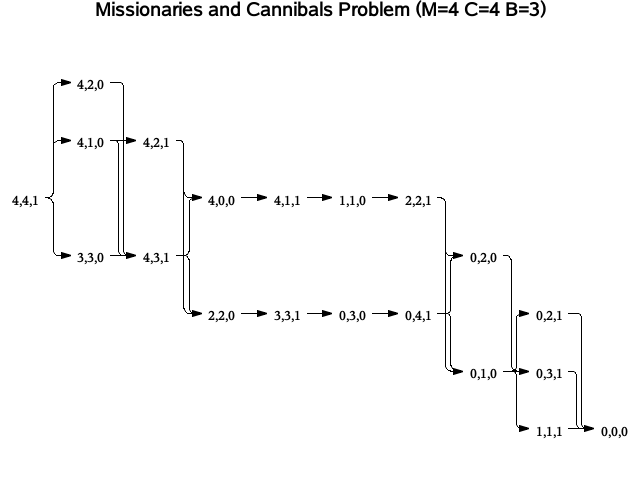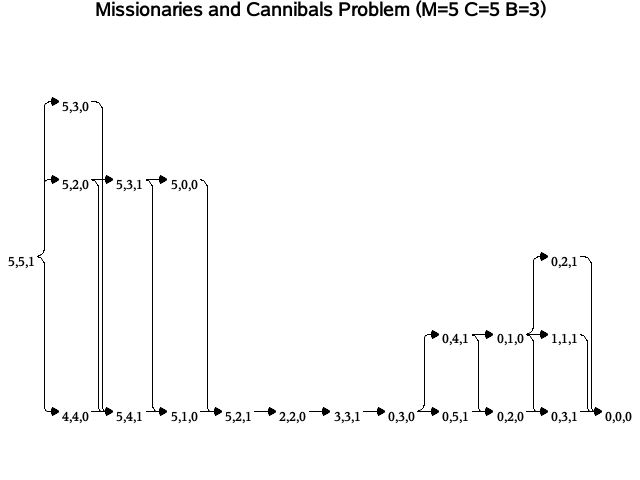For solving an upper missionaries and cannibals Problem (M=5, C=5, B=3), the step description of a solution also can be generated by SAS as below: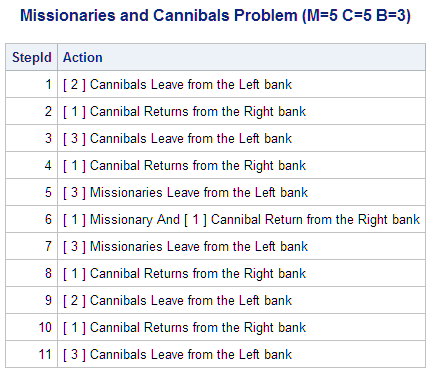In the same way, when the number of cannibals is less than that of the missionaries, such as 1 less (C=M-1), then all values of M can be solved because fewer cannibals weaken the restriction conditions.

When the capacity of boat B is greater than or equal to 4, there are solutions for all values of M if the number of missionaries and cannibals are equal (C=M). E.g., here is a list of all solutions for MCP(M=5, C=5, B=4) and the step description of a solution below:

Furthermore, the following table lists the statistics of all possible MCP solutions when M<=16, C=M, B=1 to 6. The SolutionsNum column indicates the number of solutions while MinTrips and MaxTrips indicate the minimum and maximum trips needed, respectively. The DistinctTripsLength column indicates whether the number of trips is variable; the distinct trips length is either 1 or 2.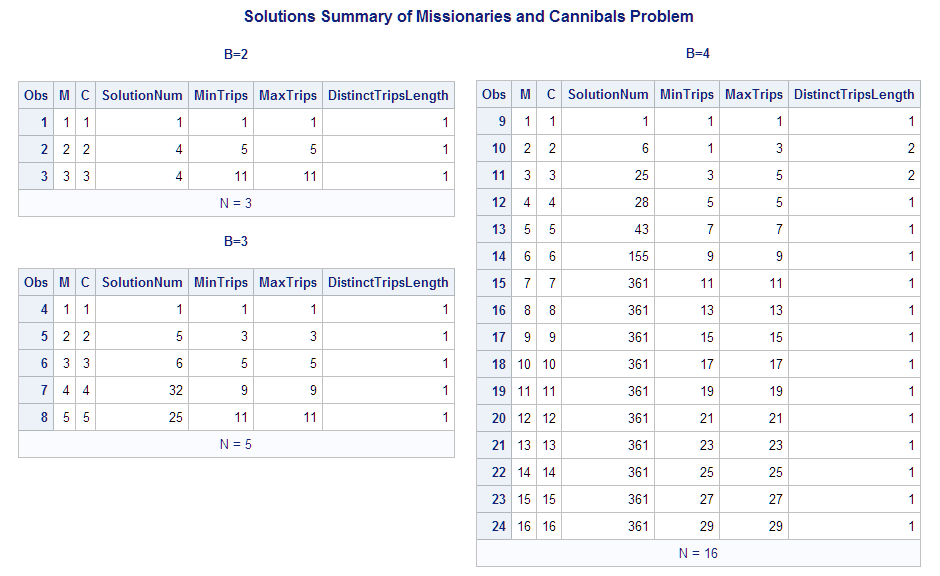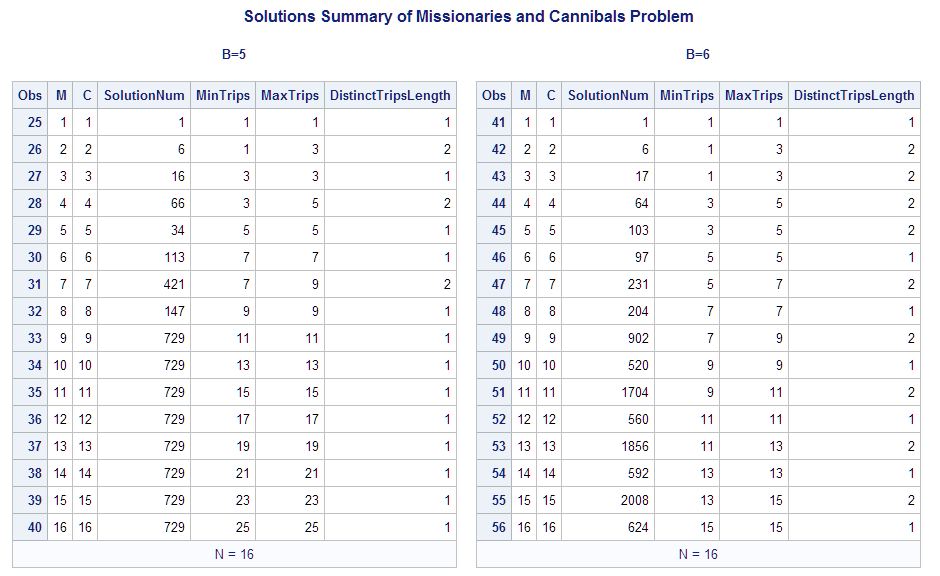The maximum number of trips across the river is not monotonically increasing, they show the following correlation. And when other conditions are the same, B=4 requires the greatest number of trips if M>=6.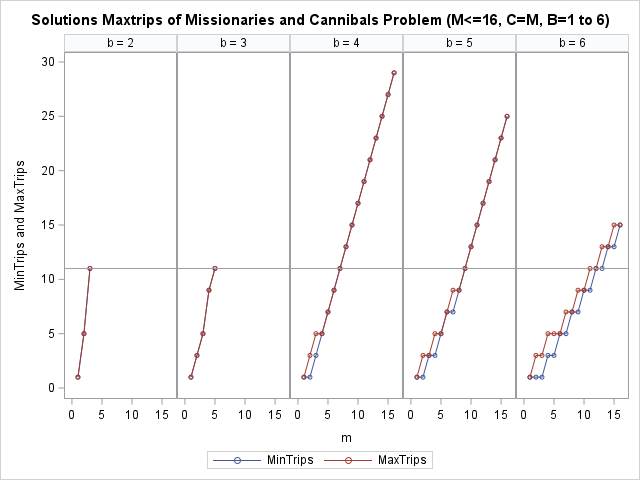The first case with a variable number of trips is MCP(M=2, C=2, B=4), it has 6 solutions with 1 or 3 trips; the second case with a variable number of trips is MCP(M=3, C=3, B=4), it has 25 solutions with 3 or 5 trips, you can see more details below:

### Summary and more resources

We have described how to solve the classic Missionaries and Cannibals problem with SAS, visualized solutions with SAS NETDRAW procedure and generated steps description automatically for any MCP problem. The statistics of all possible MCP solutions when M<=16 proved that MCP(M=3, C=3, B=2) is the only case that conforms to Theorem 4. This is just one example of how powerful SAS can be for problem-solving and data visualization. For more examples, please check out some of my other articles:

Solve the Missionaries and Cannibals Problem with SAS was published on SAS Users.